### Total 107 Videos found in Category "Algebra 1 Examples"

Page: 1 2 3
Title
 1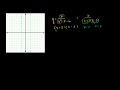A Third Example of Graphing a Rational Function 2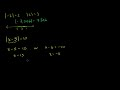Absolute Value Equations 3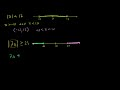Absolute Value Inequalities 4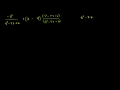Adding Rational Expressions Example 1 5Adding Rational Expressions Example 2 6Adding Rational Expressions Example 3 7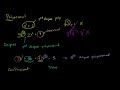Addition and Subtraction of Polynomials 8Addition of Rational Numbers 9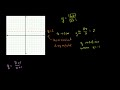Another Rational Function Graph Example 10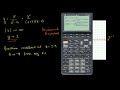Asymptotes of Rational Functions 11Average or Central Tendency: Arithmetic Mean, Median, and Mode 12Box-and-whisker Plot 13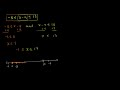Compound Inequalities 14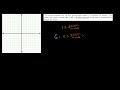Direct Variation Models 15Discriminant of Quadratic Equations 16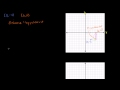Distance Formula 17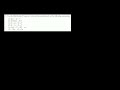Distributive Property Example 1 18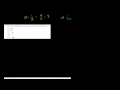Division of Rational Numbers 19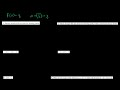Domain and Range of a Function 20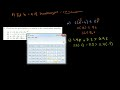Equations and Inequalities 21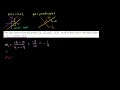Equations of Parallel and Perpendicular Lines 22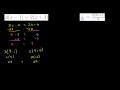Equations with Variables on Both Sides 23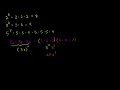Exponent Properties Involving Products 24Exponent Properties Involving Quotients 25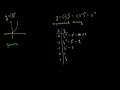Exponential Decay Functions 26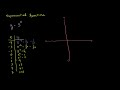Exponential Growth Functions 27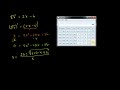Extraneous Solutions to Radical Equations 28Factor by Grouping and Factoring Completely 29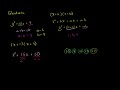Factoring Quadratic Expressions 30Factoring Special Products 31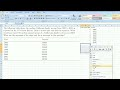Fitting a Line to Data 32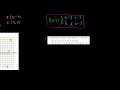Functions as Graphs 33Geometric Sequences (Introduction) 34Graphing Inequalities 35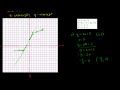Graphing Using Intercepts 36Graphs of Linear Equations 37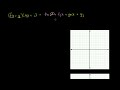Graphs of Quadratic Functions 38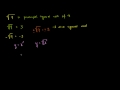Graphs of Square Root Functions 39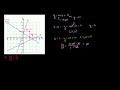Graphs Using Slope-Intercept Form 40Histograms 41How to Rationalize a Denominator 42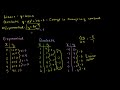Identifying Exponential Models 43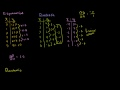Identifying Quadratic Models 44Inequalities Using Addition and Subtraction 45Inequalities Using Multiplication and Division 46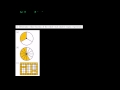Integers and Rational Numbers 47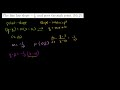Linear Equations in Point Slope Form 48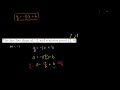Linear Equations in Slope Intercept Form 49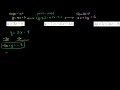Linear Equations in Standard Form 50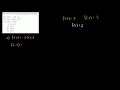Linear Function Graphs

Say and share some thing about these videos...